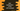# Dart list : Explanation with example### Introduction :

List or array of similar items is used in all programming languages.In dart, this is represented by array or commonly known as lists. List is an ordered group of objects. The index of the list starts from 0.So, the index of the first element is 0,second element is 1 etc. Using this index,we can access any value for a specific position in the list.Creating a simple looks like as below :

``````main(List args) {
var myList = [1,2,3];
print(myList);
}
``````

So, the list literal in dart looks like same as javascript array literal. We can add new elements to the list using add() method. It append items to the end of the list :

``````main(List args) {
var myList = [1,2,3];
print(myList);
}
``````

It will print [1,2,3,4] as output. Later,we will learn different methods that can be used with list.

### Fixed length and Growable list :

As the name indicates, a fixed length list has fixed number of elements. We cann’t append any new items to the list. A Growable list’s size is dynamic,i.e. we can add as many items we want. To create a fixed length list, the main idea is to assign list size while creating. Means, if you are creating one list like new List(max_size), then it will always have maximum size of max_size.One error will be thrown if we will try to add new element to the list. e.g. :

``````main(List args) {
List myList = new List(4);
}
``````

Here, we have declared the size of list myList as 4. So, while we are trying to add one new number to the list on the next line,it will first check the type of the list and since it is a fixed-size list, one error like below will be thrown :

``````Unsupported operation: Cannot add to a fixed-length list
``````

We can at max add four elements to the list :

``````main(List args) {
List myList = new List(4);
myList = 1;
myList = 2;
myList = 3;
myList = 4;
print(myList);
}
``````

It will work because we are accessing the index 0,1,2 and 3 of the list and assigning them values. It will print [1,2,3,4] as the output.

But unlike fixed-length lists, growable lists are dynamic in size. We don’t need to define their size at the declaration time, and we can add any number of items we want to add.

``````main(List args) {
List myList_1 = new List();
List myList_2 = [];
List myList_3 = [1,2];

myList_2.length = 2;
myList_2 = 3;
myList_2 = 4;

myList_3.length = 1;
myList_3 = 5;

print(myList_1);
print(myList_2);
print(myList_3);
}
``````

Output :

``````[1, 2]
[3, 4]
[5, 6]
``````

In this example, we have created 3 different lists and all are growable lists. For the first list myList_1, we are not assigning maximum length. We are just adding elements using add() method and the size is keep increasing. For the second list myList_2, first, we set the length as 2 and then set values for both position. And, for the last one , first we set the length as 1, assign value for the first element and then add one more element using add(). The length was previously 1,but after add(), it became 2.Similarly, we can add more elements using add().

### Properties of Dart list :

1. first : It returns the first element of the list.
2. last : It returns the last element of the list.
3. length : It returns the total number of objects in a list.
4. reversed : It returns one iterable of the objects in the list in reverse order.
5. hashCode : hash code of the object.
6. isEmpty : Returns one boolean if the list is empty or not.
7. isNotEmpty : Returns one boolean if the list has elements or empty.
8. single : Checks that the list has only one element and returns the element.